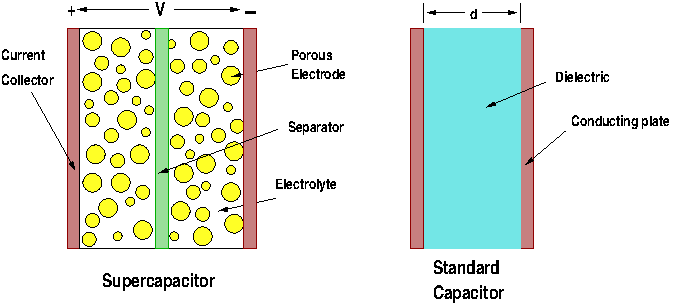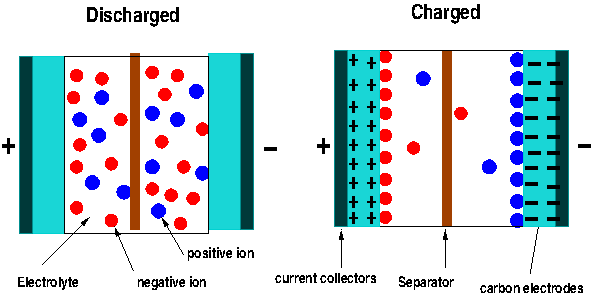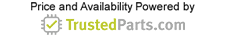Consumer Peripherals

# Batteries, Supercapacitors and Graphene Supercapacitors

19 June 2017

For a long time now, we have been trying to develop energy storage devices to keep track of the rapid development of electronic devices that need fast charging and large charges. Batteries are the best devices we have to store large amounts of energy, but they are not practical in the era of small high tech devices like smart phones. For large storage we need large batteries; in addition, batteries are charged and deliver the stored energy at a very low speed because they store energy using a chemical reaction where the ions created by the reaction move slowly toward the electrodes. This is the main reason electrically powered vehicle are still in the early stages of being a common consumer product as we thought over twenty years ago. Nowadays, a fully charged car, like the Tesla Model S, can travel up to 250 miles on a single charge, whereas to charge it takes more than 40 hours using the 120 volt socket at home.

Capacitors can charge and discharge at a very high speed, but the amount of charge that can be stored is much less than batteries. On the other hand, supercapacitors, or ultracapacitors, are a special type, different from normal capacitors that are designed to store an enormous amount of charge. Their physical structure is similar to normal capacitors only differing in the type of materials used to manufacture them. Standard supercapacitors, however, are very expensive to produce. This is the reason they are used in few applications nowadays. Recently, however, the discovery that graphene can be used to replace the expensive material in supercapacitors, and the discovery of inexpensive methods to fabricate graphene, lead to new possibilities for cheap energy storage devices with high charge and discharge rates.

Capacitors

A normal capacitor consists of two conducting (metallic) plates separated by an insulator (dielectric.) The plates can be aluminum foils, thin films metal, or any good conducting material. The dielectric between the plates is used to increase the capability of the capacitor to store charges. For a dielectric we can use glass, ceramic, air, plastic film, paper, mica and others. When a voltage is applied between the plates electrons from the plate connected to the negative terminal migrate to the plate connected to the positive terminal of the voltage source. This produces an accumulation of positive charges in one plate and negative charges in the other. The role of the insulator is to increase capacitance (C). The larger the capacitance value the more electric charges it can hold. The unit of capacitance is the farad (F). Capacitance is a function only of the geometry of the structure and the permittivity of the dielectric. It is directly proportional to both the permittivity of the dielectric and the area of the conducting plates, and inversely proportional to the separation distance of the plates.The equation to determine the value of the capacitance of a parallel plate capacitor is given by

C = eA/d farads (F)

Where A is the area of the conducting plates, d is the separation distance of the plates and e is the permittivity of the dielectric. To illustrate why a standard capacitor can't store large charges, let's assume we have a capacitor where d = 0.1 m (10 cm) and the dielectric is air (e = 8.854E-12 F/m). To have a capacitor with C= 1F, the area of the plates should be A = 1.13E10 m2.

This is over 400 square miles of area for each conducting plate! And for only one farad! To increase the capacity of capacitors we need to increase the surface area of the plates or increase the value of the dielectric, or both. Graphene capacitors fulfill these two requirements, as we will see. A general structure of supercapacitor cell and a standard capacitor is shown in Figure 1.Figure 1: An ultracapacitor cell and a standard capacitor.

Supercapacitors

These devices, also known as electric double-layer capacitors (EDLC), have two conducting plates as standard capacitors, but are coated with a very porous material known as activated carbon. This material provides a large surface area to increase the capacitance. The device is immersed in an electrolyte solution made of positive and negative ions. Supercapacitors store energy using two methods. First, when voltage is applied to the electrodes, charges build in the carbon electrodes, making one positive and the other negative. This is the typical behavior of a standard capacitor, where an electric field is created between the plates where the energy is stored. Second, once these charges are created on the electrolytes the ions in the solutions are attracted to the charged plates. The negative ions in the solution move to the positive electrode and the positive ions to the negative plate. Thus each plate ends up having two layers of charge on their surface, as is shown in Figure 2. This is the reason we call these devices double-layer capacitors. In fact, each electrode is one capacitor, and the supercapacitor is in reality two capacitors in series.Figure 2: The charging and discharging of supercapacitors.

Now, why is the capacitance of these devices much higher than the capacitance of the standard capacitors? Let’s look again at the equation of the capacitance. The capacitance, as we saw, is inversely proportional to the distance between the electrodes. The smaller the distance (separation of charges) the bigger the capacitance. In a typical capacitor the distance between the two plates is measured in terms of tens of microns – limited by the size of the dielectric (paper film or ceramic) thickness. For a supercapacitor, however, the distance between the ions and the charges in the carbon electrodes (see figure 2) is in the order of nanometers! And we have two of them.

Also, we see from the equation, the capacitance is directly proportional to the area of the electrode plates. The plate area of a typical capacitor is relatively small because is built with standard “naked” metal. Supercapacitors use activated carbon as the material of the plates and the “spongy,” corrugated material has an area 10,000 to 100,000 times bigger.

Supercapacitors and batteries

Supercapacitors are great devices, but still they can’t store as much energy as a battery. As an example, let’s look at the energy storage capability of standard capacitors in the market today. A D-type battery, for instance, has a capacitance of only 20 microfarads and it can handle as much as 300 volts. A supercapacitor of the same size has a capacitance of 300 farads, which means that at the same voltage it can (in theory) store 15 million times more energy than the D-cell capacitor. However, this is where we run into problems; the equivalent supercapacitor is able to handle no more than 2.7 volts. Higher voltages would break down the electrolyte, so realistically such a supercapacitor can store only 1,500 times the energy stored in the equivalent capacitor.

Graphene Supercapacitors

At present, supercapacitors are very expensive to produce, so the scalability in this industry is limiting the applications. It is technically possible to use ultracapacitors to drive our smart phones instead of using lithium-ion batteries. We would never have to replace the supercapacitor and, the phone would recharge very quickly, but the charge would not last long – maybe only a couple of hours.

Researchers have been looking into improving the voltage and density energy of supercapacitors by replacing the activated carbon with graphene. Why graphene? Some reasons include:

• Graphene essentially is a form of carbon with a relative surface area much larger than activated carbon.
• It can be produced at a lower cost. In a recent research effort, a team of scientists at UC Santa Clara was able to print ultracapacitor graphene electrodes using a 3-D-printing process.
• It has much higher conductivity (> 1,700 siemens per meter) by square meter than activated carbon (10 to 100 siemens per meter). This features means graphene supercapacitors can be used in very high frequency applications that currently supercapacitors cannot.
• It is lighter than activated carbon, so smaller storage devices can be produced.